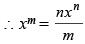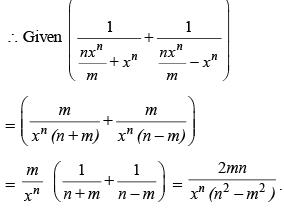Courses

# Test: Functions

## 20 Questions MCQ Test Topic-wise Past Year Questions for CAT | Test: Functions

Description
This mock test of Test: Functions for CAT helps you for every CAT entrance exam. This contains 20 Multiple Choice Questions for CAT Test: Functions (mcq) to study with solutions a complete question bank. The solved questions answers in this Test: Functions quiz give you a good mix of easy questions and tough questions. CAT students definitely take this Test: Functions exercise for a better result in the exam. You can find other Test: Functions extra questions, long questions & short questions for CAT on EduRev as well by searching above.
QUESTION: 1

### Let f be a function such that f(mn) = f(m) f(n) for every positive integers m and n. If f(1), f(2) and f(3) are positive integers, f(1) < f(2), and f(24) = 54, then f(18) equals (2019)

Solution:

Given f(mn ) = f(m) f(n) and f(24) = 54
⇒ f (24) = 2 × 3 × 3 × 3
⇒ f(2 × 12) = f(2) f(12) = f(2) f(2 × 6)
= f(2) f(2) f(6) = f(2) f(2) f(2 × 3)
= f(2) f(2) f(2) f(3) = 2 × 3 × 3 × 3
Given that f(1). f(2) and f(3) are all positive integers hence by comparison, we get
f(2) = 3 and f(3) = 2
Hear we may safely consider f(1) = 1
Now, f(18 ) = f(2) (9) = f(2) f(3 × 3)
= f(2) f(3) f(3) = 3 × 2 × 2 = 12.

QUESTION: 2

### Let f(x) = min{2x2, 52 − 5x}, where x is any positive real number. Then the maximum possible value of f(x) is (2018)

Solution:

The maximum value of f(x) will occur when
2x2 = 52 – 5x i.e. when 2x2 + 5x − 52 = 0
⇒ 2x2 + 13x − 8x − 52 = 0
⇒ (2x + 13) (x – 4) = 0 ⇒ x = – 13/2 or 4. But x is any positive real number. So, x = 4.
Hence, maximum value of f (x) = 2(42) = 32

QUESTION: 3

### If f(x + 2) = f(x) + f(x + 1) for all positive integers x, and f(11) = 91, f(15) = 617, then f(10) equals (2018)

Solution:

f(x + 2) = f(x) + f(x + 1)
f(11) = 91
Let f(12) = a
f(13) = 91 + a
f(14) = 91 + 2a
f(15) = 182 + 3a = 617
⇒ a = 145
Now, f(10) = f(12) – f(11) = 145 – 91 = 54

QUESTION: 4

The area of the closed region bounded by the equation |x| + |y| = 2 in the two-dimensional plane is

(2017)

Solution: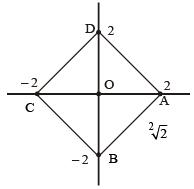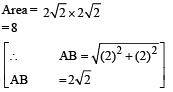QUESTION: 5

Consider two figures A and D that are defined in the co-ordinate plane. Each figure represents the graph of a certain function, as
defined below:
A: | x | - | y | = a
D :| y | = d
If the are enclosed by A and D is O. Which of the following is a possible value of (a, d):

(2016)

Solution:

The lines represented by A where a > 0 and when a < 0 are given in the following figures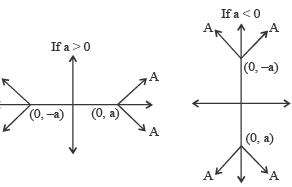The area enclosed by A and D would be zero if d < |a|. In choice (b), d = 1 and a = – 2 i.e., d < |a|.
If a > 0, then the only case when the area enclosed by A and D will be zero, is when d = 0.

QUESTION: 6

If [log10 1] + [log10 2] + [log10 4] +...+ [log10 n] = n where [x] denotes the greatest integer less than or equal to x, then

(2016)

Solution:

[log10 x] = 0, for any value of x ∈ {1, 2, ......9), ...(1)
Similarly [log10 x] = 1, for x ∈ {10, 11, 12 ... 99}...(2)
and [log10x] = 2, for
x ∈ {100, 101, 102, ... 999} ...(3)
Now consider, 1 ≤ n ≤ 9, then
[log101] + [log102] + [log103] ... [log10 n] = 0 (i.e., ≠ n)
Hence the expression given in the question cannot be satisfied.
Now consider, 10 ≤ n ≤ 99, then [log101] + [log102] ... [log10n]
From (1) and (2), the above expression becomes (0 + 0 ... 9 times) + (1 + 1 + ... (n – 9) times) = n – 9
Using the same approach, for
100 ≤  n ≤ 999, [log 101] + [log102] ... [log 10n] = 90 + 2(n – 99)
If can be seen that, only for the third case i.e., 100 ≤  n ≤  999, can the expression given in the question be satisfied.
Hence 90 + 2(n – 99) =  n
⇒ n = 198 – 90 = 108
∴ 107 ≤ n < 111.

QUESTION: 7

The coordinates of two diagonally opposite vertices of a rectangle are (4, 3) and (-4,-3). Find the number of such rectangle(s), if the other two vertices also have integral coordinates.

(2015)

Solution: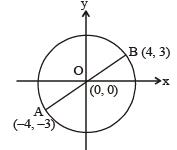Other two vertices will make two right angled triangles with AB as the common hypotenuse. So they must lie on the circle with AB as the diameter and O as the centre. Radius of that circle will be 5 units.
There will be 5 such pairs in which both the coordinates are integers.
[(5, 0), (–5, 0), [(4, 3), (4, – 3)],
[(–3, 4), (3, –4)] [(–3, –4), (3, 4)] and [(0, 5), (0, –5)]

QUESTION: 8

‘f’ is a real function such that f(x + y) = f(xy) for all real values of x and y. If f(–7) = 7, then the value of f(–49) + f(49) is

(2014)

Solution:

Let us assume f(0) = K, where 'K' is a constant.
Then, f(0 + y) = f(0.y) = f(0) = K
and  f(x + 0) = f(x.0) = f(0) = K.
This proves that the function is a constant function.
Thus, the value of
f(–49) = f (49) = 7
Hence, f(–49) + f(49) = 14.

QUESTION: 9

Let f(x) = ax2 + bx + c, where a, b and c are real numbers and a ≠ 0. If f(x) attains its maximum value at x = 2, then what is the sum of the roots of f(x) = 0?

(2013)

Solution:

The sum of the roots of ax2 + bx + c = 0 is -b/a
By differentiating we get 2ax + b = 0
ax2 + bx + c attains its maximum value at x = -b/2a
∴ -b/2a = 2 ⇒ -b/a = 4
Hence, the sum of the roots = 4.

QUESTION: 10

If f(x) = (secx + cosecx)(tanx - cotx) and π/4 < x < π/2, then f(x) lies in the range of

(2013)

Solution:

Convert into sine form this is a increasing function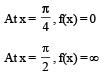Hence, f(x) lies in the range of (0, ∞)

QUESTION: 11

At how many points do the graphs of y = 1/x and y = x2 – 4 intersect each other?

(2013)

Solution:

The graphs of the two functions are shown below: If a > 0 in any parabolic function then parabola open up side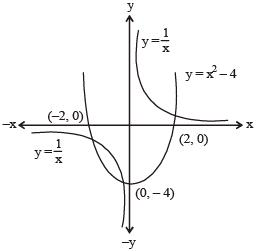From the above figure, it is obvious that the graphs of the two functions intersect at three points.

QUESTION: 12

A function F(n) is defined as F(n - 1) = 1/(2 - F(n)) for all natural numbers ‘n’. If F(1) = 2, then what is the value of [F(1)] + [F(2)] +…………+ [F(50)]?
(Here, [x] is equal to the greatest integer less than or equal to ‘x’)

(2012)

Solution:

Given that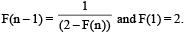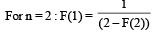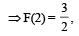Similarly, we can find the values of F(3), F (4), F (5) as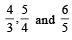respectively..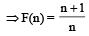From this we can say that every term except [F(1)], of the series [F(1)] + [F (2)] + .... + [F (50)] is equal to 1 as for 'n' > 0, F (n) lies between 1 and 2.
Therefore, [F (1)] + [F (2)] + .... +  [F (50)] = 51.
Hence, option (a) is the correct choice.

QUESTION: 13

A function f(x) is defined for real values of x as: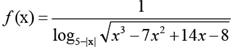What is the domain of f(x)?

(2012)

Solution: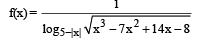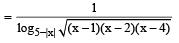Base of the logarithmic function 5 – |x| > 0 and 5 – |x| ≠ 1
So, x ∈ (–5, –4) ∪ (–4, 4) ∪ (4, 5) ...(i)
Also, (x–1) (x–2) (x–4) must be greater than zero as well
So, x ∈ (1, 2) ∪ (4, ∞) ...(ii)
Combining (i) and (ii) : x ∈ (1, 2) ∪ (4, 5)

QUESTION: 14

Let f be an injective map with domain{x, y, z} and range {1, 2, 3} such that exactly one of the following statements is correct and the remaining are false. f(x) = 1, f(y) ≠ 1, f(z) ≠ 2. The value of f –1(1) is

(2011)

Solution:

There are following three cases arise :
Case (I) :
When f (x ) = 1, is correct
then f (y ) = 1 and f (z ) = 2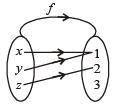clearly the mapping f is not injective (i.e., not one-one) Hence this case is not possible.
Case (II) :
When f (y) ≠ 1, is correct
then f (x) ≠ 1 and f (z) = 2
Hence z mapped to 2 but x and y or mapped to 2 or 3 or one of them mapped to 2 and the other mapped to 3.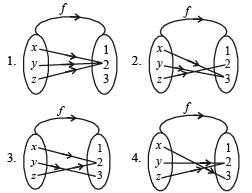Clearly in all the above 4 sub-cases, we see that the mapping f is not injective (i.e. not one-one). Hence, this case is not possible.
Case (III) :
If f (z) ≠ 2, is correct
then f(x) ≠  1 and f (y) =1
Hence y mapped 1 but x mapped 2 or 3
Whereas y mapped 1 or 3.
The possible four mapping are as follows :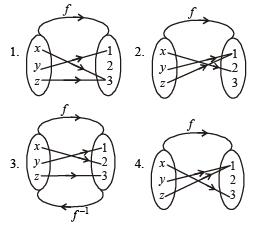Clearly in sub case (c), the mapping is injective (i.e., one-one). Hence this case is possible and f -1 (1) = y

QUESTION: 15

The shaded portion of figure shows the graph of which of the following?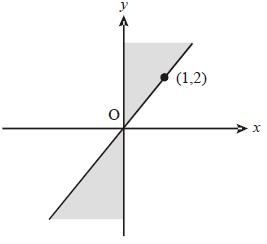(2011)

Solution:

Each of the answer choices in the form of the product of two factors on the left and a “≥ 0” or “ ≤ 0” on the right.
The product will be negative when the two factors have opposite signs, and it will be positive when the factors have the same sign. Choice (a), for example, has a “≥0”, so you’ll be looking for other factors to have the same sign.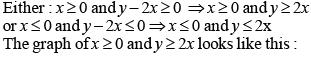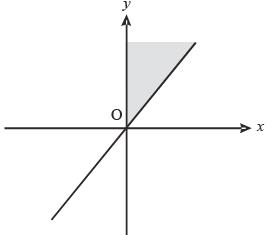The graph of x ≤ 0 and y ≤ 2x looks like this.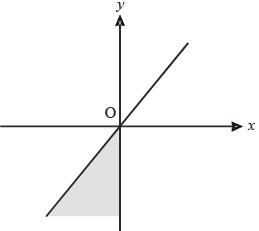Together, they make the graph in the figure.

QUESTION: 16

When ‘2’ is added to each of the three roots of x3 – Ax2 + Bx – C = 0, we get the roots of x3 + Px2 + Qx – 18 = 0. A, B, C, P and Qare all non-zero real numbers. What is the value of (4A + 2B + C)?

(2011)

Solution:

Let the roots of the equation x3 – Ax2 + Bx – C = 0 be α, β, γ respectively.
So the roots of x3 + Px2 + Qx – 18 = 0 will be α + 2, β + 2, γ + 2.
(α +2)(β +2)(γ +2) = 18
⇒ 4(α + β + γ) + 2 (αβ + βγ +  γα) + αβγ + 8 =18
⇒ 4A + 2B + C = 10

QUESTION: 17

If three positive real numbers a, b and c (c > a) are in Harmonic Progression, then log (a + c) + log (a – 2b + c) is equal to:

(2010)

Solution:

Since a, b, c are in H.P.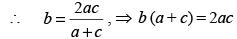Now log (a + c) + log (a – 2b + c)
= log [(a + c){(a + c) – 2b}]
= log [(a + c)2 – 2b (a + c)]
= log [(a + c)2 – 2 × 2ac]
= log (a – c)2 or log (c – a)2
= 2 log (a – c) or 2 log (c – a)
∴ log (a + c) + log (a – 2b + c) = 2 log (c – a)

QUESTION: 18

There are three coplanar parallel lines. If any p points are taken on each of the lines, then find the maximum number of triangles with the vertices of these points.

(2010)

Solution:

The number of triangles with vertices on different lines
= PC× PC1 x PC= p3
The number of triangles with 2 vertices on one line and the third vertex on any one of the other two lines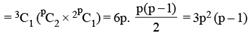∴ the required number of triangles = P3 + 3P2(P - 1)
= 4p3 – 3p2 = p2 (4p – 3)
(The work “maximum” shows that no selection of points from each of the three lines are collinear).

QUESTION: 19

If f (x + y/8, x - y/8) = xy, then f (m, n) + f (n, m) = 0

(2010)

Solution: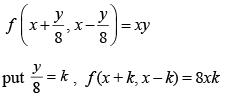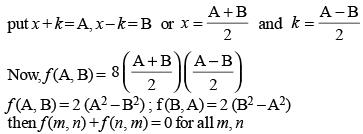QUESTION: 20

If mxm – nxn = 0, then what is the value of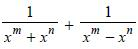in terms of xn ?

(2010)

Solution:

mxm = nxn